# Numerical and experimental investigation on the transient behavior of an earth air heat exchanger in continuous operation mode

Numerical and experimental investigation on the transient behavior of an earth air heat exchanger in continuous operation mode

Yousef Belloufi*Abdelhafid Brima Sakina Zerouali Rachid Atmani | Faris Aissaoui Amar Rouag Noureddine Moummi

Laboratoire de Génie Mécanique LGM, BP 145.07000 University of Biskra, Algeria

Laboratoire de Modélisation des Systèmes Energétiques LMSE, BP 145.07000 University of Biskra, Algeria

Laboratoire de Génie Energétique et Matériaux LGEM, BP 145.07000 University of Biskra, Algeria

Corresponding Author Email:
yousef_belloufi@yahoo.fr
Page:
279-288
|
DOI:
https://doi.org/10.18280/ijht.350208
|
Accepted:
|
Published:
30 June 2017
| Citation

OPEN ACCESS

Abstract:

The main objective of the present study is to investigate the thermal performances of an earth air heat exchanger (EAHE)under transient conditions in cooling mode. This work was performed experimentally in the University of Biskra, Algeria.For that, a PVC pipe of 53.16 m long and 110 mm diameter buried at 3 m depth is used. Tests of the experimental setup were carried out in continuous operation mode for 71 hours with high inlet temperatures. Besides, a mathematical model was presented for EAHE by using the finite differences method applying the energy balance equations with forced convection. In addition of the horizontal portion on soil surface, the two vertical portions of 3m each one connected to the horizontal portion were considered in the modeling in order to present a fairly complete study of the EAHE. It was found that the continuous operation mode have no remarkable effect on the outlet air temperature and thus on the EAHE performances during all 71 hours. Results of the theoretical predictions were validated with the measured air temperatures along the EAHE and showed satisfactory qualitative and quantitative agreement.

Keywords:

earth air heat exchanger, cooling mode, continuous operation mode, thermal comfort

1. Introduction

In the last decades, the world is experiencing of high consumption of electric power necessary to the air conditioning, especially in hot regions during the hot period. To reduce this consumption, we can find clean, safe and inexpensive alternative sources for air conditioning, like  earth air heat exchangers (EAHE). The idea of using earth as a heat sink was known in ancient times. In about 3000 B.C., Iranian architects used wind towers and underground air tunnels for passive cooling [1-4]. The main advantages of EAHE system are its simplicity, high cooling and pre-heating potential, low operational and maintenance costs, saving of fossil fuels and related emissions  .

This paper examines the air conditioning tools using an EAHE (called Canadian wells or Provencal wells). It is a ventilation system designed to preheat or refresh the air using the thermal inertia of the soil. The air aspirated from the outside transits by a buried pipe at a well-defined depth on a distance L, recovering during its path the soil energy (to heat or to cool), what allows a preheating or refreshment of the building according to the season (winter or summer).

Several experimental and theoretical researchers have developed the EAHE in the literature.

Trombe et al.  realized their experimentation in the south of France on an individual house; they noticed that the outside air can be conditioned even with very high temperatures. The experimental research of Thanu et al.  on EAHE for the thermal comfort of a building indicates that the system works and possesses a high efficiency during the summer period. Ghosal et al [8, 9] presented the modeling of an earth to air heat exchanger with a greenhouse. Shukla et al.  developed a thermal model for heating of greenhouse by using different combinations of inner thermal curtain, an EAHE and geothermal heating. Some authors [11-16] have developed theoretical studies with different analytical models of the horizontal heat exchanger on the soil surface. They concluded that in order to increase the performances of the EAHE, it is required to take into account the following parameters: i) the length and diameter of the pipe, ii) the burial depth of the underground heat exchanger and iii) the thermal properties of the soil namely (thermal conductivity λsoil and specific heat Cp). Mihalakakou et al. , Lee and Strand  presented a parametric study to investigate the effect of pipe length, pipe radius, air flow rate and depth of the buried pipe on the overall performance of the EAHE under various conditions in cooling mode. Brum et al. , who have developed a new numerical approach (called reduced model) to obtain the fluid dynamics and thermal behavior of the EAHE, their results are validated with literary archives. Vaz et al.  presented an experimental study of an annual cycle realized in the south of Brazil. A numerical solution of the conservation equations is performed with commercial code 'FLUENT' based on the finite volume method. Thiers et al.  considered that only part of the soil is influenced by the heat exchanger, and take into account the interaction of several tubes by comparing the distance between them and the depth of penetration defined by Hollmuller . Barakat et al  developed a Transient one-dimensional model for predicting the impact of main geometrical and dynamical parameters including tube length, inner tube diameter and inlet air velocity on the performance of the EAHE system. Ozgener et al  designed and installed an EAHE in Turkey for the determination and evaluation of thermal properties of the heat exchanger. An Average total heat exchanger thermal resistance was estimated to be 0.021 K-m/W as a constant value under steady state condition. Mathur et al  studied soil thermal saturation and self recovery ability under intermittent and continuous operation modes of EAHE. They were developed a numerical model using ANSYS 14.5, its results have been validated experimentally through a full scale setup. They found that the soil temperature can be recovered in both intermittent and continuous operation modes by employing natural heat conduction and convection (where inlet air temperature in night hours is lower than soil temperature). Vaz et al  concluded that the soil temperature around the buried pipe gets affected by the continuous operation of EAHE. They developed a correlation between the soil and buried pipe temperature variation using linear regression model. Bansal et al  studied the effect of soil thermal conductivity and time period of continuous operation of EAHE on its thermal performance. In this way, a three different types of soil thermal conductivities of 0.52 w/m°c, 2 w/m°c and 4 w/m°c are compared. It was concluded that the thermal performance of EAHE deteriorates with prolonged operation and soil having poor thermal conductivity. Mathur et al  investigated thermal performance of an EAHE under transient conditions for three different soil thermal conductivities considering three operating modes. In mode 1 (12 h of continuous operation), mode 2 (cycle of 12 h of intermittent operation i.e 60 min ON and 20 min OFF) and mode 3 (cycle of 12 h of intermittent operation i.e 60 min ON and 40 min OFF). The outlet air temperatures have been numerically determined using CFD software Fluent V 6.3. it was concluded that EAHE system with higher soil thermal conductivity (1.28 w/m k) can be operated continuously (mode 1). Bansal et al  introduced a new concept named ‘Derating Factor’ for evaluating thermal performance of EAHE under transient operation conditions using experimental and CFD modeling with Fluent software. The derating Factor is affected by air flow velocity inside the EAHE, pipe geometry, ambient conditions and type of operation (intermittent or continuous). They concluded that for better thermal performance, it was advisable to operate the EAHE system in soil with higher thermal conductivity. Yang et al  evaluated the thermal performance of EAHE, they proposed an analytical model in periodically fluctuating thermal environment without using ‘penetration radius’ to confine the distance of heat diffusion in soil. The presented model costs much less time than CFD simulation and provides solutions with high accuracy. It was found that the buried depth, pipe length and mass flow rate are important parameters for evaluating the performance of EAHE. Chel et al  evaluated the dynamic thermal performance if building integrated with water heat exchanger (WAHE) and EAHE by using transient system simulation (TRNSYS). Their results show that WAHE and EAHE had a reduction of the annual heating consumption of 66% and 7% respectively. Hatraf et al  presented a parametric study for designing an EAHE and evaluating its thermal performance. They showed that the efficiency of the EAHE depends of on buried depth of pipe and pipe dimensions. The pipe material exceptionally does not affect the thermal performance of the EAHE. Misra et al  investigated experimentally the thermal performance of hybrid EAHE system in four different modes by integrating active and passive systems. Inside the room, a temperature of 25°C is remained constant during each mode. Mode 1 (air conditioner alone supplies the conditioned air to the room without functioning EAHE), mode 2 (air conditioner supplies conditioned air to room and 100% conditioned air from EAHE is also delivered directly to the room), mode 3 (like mode 2, but exceptionally the EAHE is functioned for cooling the condenser tubes of air conditioner) and mode 4 (air conditioner supplies the conditioned air to the room, 50% conditioned air from EAHE is injected to the room directly and remaining 50% air is used for condenser cooling). It was found that the third mode reduced 18% of electrical energy consumption in comparison with first mode and an increasing of 16% in electrical energy consumption in fourth mode as compared to the first mode.

The aim of this study is to clarify the effect of the continuous operation mode, soil thermal conductivity and air flow velocity on the thermal performance of the EAHE. Unlike previous studies which examined the horizontal portion of the EAHE, our contribution in this work is to present a fairly complete theoretical study in all parts of the EAHE (verticals and horizontal portions) Fig. 1. The experimental measurements were realized in the region of Biskra for three days in continuous operation mode and used to validate the proposed numerical model. The region of Biskra is typical climate for air cooling in hot period. Where the air temperature in this arid region recorded in July and August with an afternoon average temperature of 47°C.

2. Modeling

The following assumptions are used to simplify the modeling: i) From a defined depth δ the temperatures remain constant in the soil, ii) soil far than the EAHE keeps a constant temperature during the heat exchange, iii) convective heat transfer coefficient is constant along the pipe, iv) longitudinal conduction and soil moisture are neglected and v) perfect contact between the pipe and the soil surrounding.

We divide the EAHE into three portions (vertical portion I, horizontal portion II and vertical portion III) as mentioned in the Fig.1.

## 1.png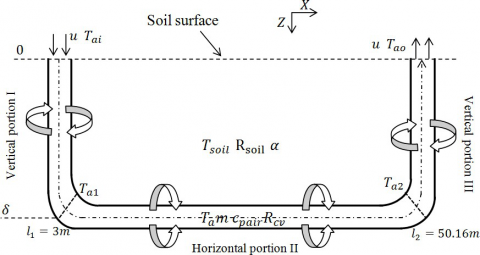Figure 1. Descriptive scheme of the EAHE.

2.1 Soil temperature around the EAHE

The soil temperature around vertical portions I and III in the vertical coordinate is based on the resolution of heat equation in unsteady state .

$\frac{\partial^{2} T_{\text {soil}}}{\partial z^{2}}=\frac{1}{\alpha} \frac{\partial T_{\text {soil}}}{\partial t}$    (1)

The following boundary conditions are used for solvingeq.(1)

$T_{\operatorname{soil}}(z=0)=T_{p}$    (2)

$T_{\text {soil}}(z=\delta)=T_{i}$    (3)

$T_{\text {soil}}(t=0)=T_{i}(z)=a z+b$    (4)

The unknown constants a and b can be determined by substituting the boundary conditions (2) and (3) in eq. (4).When is the depth of buried pipe, is the soil temperature at depth and is the soil surface temperature, where we ensure the heat flow continuity between the soil and the ambient air to define it .

$T_{p}=\frac{\lambda_{\text {soil}}}{\lambda_{\text {soil}}-\delta h} T_{i}-\frac{\delta h}{\lambda_{\text {soil}}-\delta h} T_{a i}$    (5)

The distribution of the soil temperature eq. (6) is then obtained by imposing the boundary conditions (2), (3) and (4).

$T_{\text {soil}}(z)=\left(T_{p}-T_{i}(z)\right)\left(1-\operatorname{erf}\left(\frac{z}{2 \sqrt{\alpha t}}\right)\right)+T_{i}(z)$    (6)

2.2 Temperature of the air along the EAHE

The portions I and III are buried vertically to the soil surface, it assembled to the horizontal portion II Fig. 1 which is buried sufficiently deep in the soil, to keep the soil temperature Tsoil constant and less sensitive to external climatic conditions. The energy balance equations between two sections distant of inside the portions I, II and III Fig.2 can be written as follow

$m c_{p a i r} \frac{D T_{a}}{D t}=q_{1}-q_{2}-q_{3}$    (7)

$m c_{p a i r} \frac{D T_{a}}{D t}=q_{1}-q_{2}+q_{3}^{\prime}$    (8)

where eq. (7) represnts to the energy balance equation of the portions I and II Figs. 2a and 2b respectively and eq. (8)represnts to the vertical portion III Fig. 2c where q3 = - q3so eqs. (7) and (8) have the same result. From eqs. (7) and (8),we obtain

$m c_{p a i r}\left(\frac{\partial T_{a}}{\partial t}+u \frac{\partial T_{a}}{\partial z}\right)=-\left.\lambda_{a i r} s \frac{\partial T_{a}}{\partial z}\right|_{l}+\left.\lambda_{a i r} s \frac{\partial T_{a}}{\partial z}\right|_{l+\Delta l}+\frac{\left(T_{s o i l}-T_{a}\right)}{R_{\text {total}}}$    (9)

where u is the air velocity inside the pipe,$s=\pi \cdot r_{1}^{2}$ is the internal section of the pipe.

## 2.jpg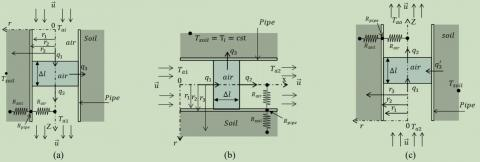Figure 2. Descriptive scheme of the EAHE: a) vertical portion I, b) horizontal portion II, c) vertical portion III.

The total thermal resistance (Rtotal) is composed of the soil conductive resistance (Rsoil), the pipe resistance (Rpipe) and the air convective resistance (Rcv).

$R_{t o t a l}=R_{s o i l}+R_{p i p e}+R_{c v}$    (10)

We know that $\mathbf{r}_{3}(\mathrm{t})=\sqrt{\frac{\alpha . t}{\pi}}$ is the adiabatic layer radius of the soil surrounding the pipe and h = (Nu k)/2 r1 is the convective heat transfer coefficient of the flowing air inside the EAHE, where Nu = 0.023 Re0.8 Pr0.3  is the Nusselt number and Re = ρv/µ is the Reynolds number.Taking into account the fact that convection dominates conduction, eq. (9) reduces to.

$\rho s c_{p a i r}\left(\frac{\partial T_{a}}{\partial t}+u \frac{\partial T_{a}}{\partial z}\right)=\frac{\left(T_{s o i l}-T_{a}\right)}{R_{i to t a l}}$    (11)

Ritotal is the total thermal resistance per unit length.

$\frac{\partial T_{a}}{\partial t}=-u \frac{\partial T_{a}}{\partial z}+\frac{\left(T_{\text {soil}}-T_{a}\right)}{\gamma}$    (12)

with $\gamma=\rho \pi r_{l}^{2} c_{\text {pair}} R_{\text {itotal}}$

Choosing the following initial conditions for all parts of EAHE in order to solve eq. (12).Ta (L = 0) = Tai, which is the measured air temperature at the inlet of EAHE.

Ta (L = l1) = Ta1, which is the air temperature at the outlet of vertical portion I.

Ta (L = l2) = Ta2, which is the air temperature at the inlet of vertical portion III.

Ta (t = 0) = Tsoil,[36-38]

To solve eq. (12), implicit finite differences used to discretize both space and time domains eq. (13). Space domain discretizes to some elements Nx= 5316 with an element size Δx= 0.01m and time domain discretizes to a number of time steps Nt with a time step Δt= 15 sec. Fig. (3)presents the EAHE configuration and the discrete domain.

## 3.png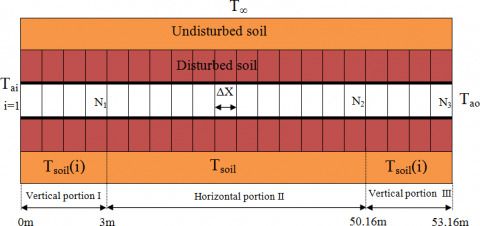Figure 3. Discretization of EAHE domain.

$\frac{1}{\Delta t}\left[T_{i, j+1}-T_{i, j}\right]=-\frac{u}{2 \Delta l}\left[T_{i+1, j+1}-T_{i-1, j+1}\right]-\frac{1}{\gamma} T_{i, j+1}+\frac{T_{s o i l}}{\gamma}$    (13)

$T_{i, j}+\frac{\Delta t}{\gamma} T_{s o i l}=-\frac{u \Delta t}{2 \Delta l} T_{i-1, j+1}+\left(\frac{\Delta t}{\gamma}+1\right) T_{i, j+1}+\frac{u \Delta t}{2 \Delta l} T_{i+1, j+1}$    (14)

The discretized form in eq. (14) was implemented in FORTRAN language where Thomas method was used to find the air temperatures in all portions of the EAHE.

The soil temperature Tsoil is considered constant in horizontal portion II and variable in vertical portions I and III eq. (6).

2.3 Derating factor

Thermal performance of EAHE is basically defined as the amount of cooling which can be produced. EAHE operating under steady state condition is taken as reference case for comparing the thermal performance of EAHE is transient conditions. Temperature drops obtained under transient conditions are used to determine the derating factor which is known as the ratio of deterioration in thermal performance under transient conditions to the thermal performance for steady state condition. Derating factor can be written as follows :

$D F=\frac{\left(T_{\text {inlet}}-T_{\text {outlet}}\right)_{\text {transient} \text {state}}}{\left(T_{\text {inlet}}-T_{\text {outlet}}\right)_{\text {steady state}}}$    (15)

2.4 EAHE thermal efficiency

The efficiency of the EAHE is defined as the ratio of air temperature drop and temperature difference between soil and inlet air temperature:

$\mathcal{E}=\frac{\left(T_{\text {outlet}}-T_{\text {inlet}}\right)}{\left(T_{\text {soil}}-T_{\text {inlet}}\right)}$    (16)

3. Experimental Setup

Soil temperature depends on several parameters, such as: the soil nature, the thermal conductivity, the heat quantity absorbed by the soil from the solar radiation and the ambient temperature. In the region of Biskra it is known that at a depth of 3m, the soil temperature remains fairly constant and less sensitive to external climatic conditions .

This work (fig .4) has been performed in the Laboratory of Mechanical Engineering LGM at Biskra University (latitude of 34°48' N, longitude of 5°44'E). The temperatures of the air were measured at distances of 0, 3.63 m, 7.69 m, 11.73 m,16.04 m, 20.07 m, 24.12 m, 26.37 m, 29.07 m, 33.10 m, 37.01 m, 38.86 m, 40.82 m, 45.10 m, 48.80 m(the outlet of horizontal portion II) and 53.16 m (the outlet of vertical portion III and EAHE) from the pipe entrance. Fig. 5 illustrates the location of thermocouples in the buried pipe. The air temperatures inside the EAHE were recorded every 15 mins during three days in continuous operation mode in August 2013, and presented in Tables 2, 3 and 4.

The EAHE shown in Fig. 4 consists of one PVC pipe of110 mm diameter and a total length of 53.16 m, the serpentine pipe buried at 3 m depth, with 2 m spacing in 2% slope. It occupies an area of 85 m². A sink is provided at the outlet of the horizontal portion II to evacuate the condensed water. A central acquisition unit provided by National Instrument,connected by 16 RTD temperature sensors to measure the air temperature along the buried pipe. The air velocity inside the pipe is managed by a volumetric flow rate extractor, this latter have low electric power consumption (120 W). The main characteristics of the system and the technical characteristics of measuring instruments are reported in Tables 1 and 2 respectively.

## 4.pngFigure 4. General view of the EAHE.

## 5.png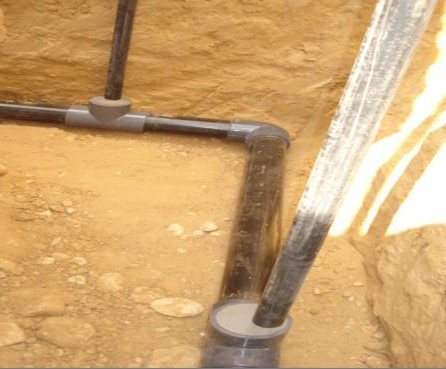Figure 5. Location of RTD temperature sensors in the buried pipe.

Table 1. Various system parameters.

 System Value Soil temperature at depth δ (Ti) 26°C Depth of buried pipe (δ) 3 m Air specific heat cpair 1000 J/(Kg.°C) Air density (ρ) 1.2 Kg/m3 Soil thermal conductivity (λsoil) 1.25 W/(m.°C) Thermal conductivity of pipe (λp 0.17 W/(m.°C) Air velocity inside the pipe (u) 3.5 m/s Inner radius of the pipe (r1) 55 mm Outer radius of the pipe (r2) 57.5 mm

Table 2. Technical characteristics of measuring instruments.

 Instrument Measuring   range Accuracy Resolution RTD temperature sensors -50 to 200°C ------ 10-5 °C propeller anemometer LV100 From 0.3 m/s to 35 m/s From 0.3 to 3 m/s : ± 3 % of reading ± 0.1 m/s From 3,1 to 35 m/s : ± 1% of reading ±   0.3 m/s 0,01 m/s     0,1 m/s

Table 3. Variation of air temperature along the EAHE (04-05/08/2013).

 Length of pipe (m) 10 :30 12 :30 14 :30 15 :15 16 :30 18 :30 20 :30 01:30 05:30 After 1h After 3h After 5h After 5:45h After 7h After 9h After 11h After 16h After 20h inlet 36.31 39.32 41.27 43.56 42.39 41.80 38.34 34.25 32.13 3.63 35.98 38.25 39.84 41.58 40.98 40.42 37.26 33.95 32.26 7.69 33.91 35.50 36.65 37.71 37.61 37.39 35.30 33.00 31.79 11.73 32.13 33.23 34.06 34.63 34.80 34.86 33.68 32.16 31.38 16.04 31.37 32.26 32.98 33.50 33.59 33.70 32.72 31.59 30.94 20.07 30.71 31.38 31.94 32.33 32.47 32.61 31.92 31.12 30.64 24.12 29.87 30.36 30.79 31.05 31.18 31.34 30.92 30.42 30.09 26.37 29.72 30.13 30.50 30.71 30.84 31.01 30.67 30.27 30.00 29.07 29.52 29.87 30.17 30.33 30.45 30.61 30.37 30.09 29.89 33.10 29.12 29.40 29.65 29.78 29.88 30.02 29.84 29.67 29.53 37.01 28.64 28.84 29.03 29.12 29.20 29.33 29.23 29.15 29.07 38.86 28.45 28.63 28.80 28.87 28.95 29.08 28.99 28.94 28.87 40.82 28.28 28.45 28.60 28.67 28.74 28.86 28.79 28.76 28.71 45.10 27.91 28.00 28.10 28.13 28.18 28.25 28.23 28.27 28.27 48.80 27.77 27.87 27.95 27.99 28.04 28.12 28.09 28.13 28.13 53.16 30.24 30.19 30.29 30.36 30.52 30.69 30.76 30.29 29.98

Table 4. Variation of air temperature along the EAHE (05-06/08/2013).

 Length of pipe (m) 10 :30 12 :30 14 :30 15 :45 16 :30 18 :30 20 :30 01:30 05:30 After 25h After 27h After 29h After 30:15h After 31h After 33h After 35h After 40h After 44h inlet 38.53 41.43 42.32 43.92 42.92 41.51 38.42 33.60 29.71 3.63 37.80 39.62 40.84 42.01 41.55 40.22 37.42 33.54 30.45 7.69 35.45 36.42 37.54 38.22 38.13 37.49 35.61 32.91 30.71 11.73 33.47 34.01 34.84 35.23 35.36 35.17 34.05 32.31 30.88 16.04 32.65 32.93 33.71 34.02 34.15 34.05 33.14 31.79 30.59 20.07 31.87 31.99 32.63 32.87 32.98 33.01 32.35 31.38 30.46 24.12 30.89 30.92 31.39 31.55 31.66 31.76 31.34 30.71 30.07 26.37 30.65 30.66 31.06 31.20 31.29 31.41 31.07 30.57 30.04 29.07 30.39 30.35 30.70 30.80 30.89 31.01 30.76 30.40 29.97 33.10 29.90 29.85 30.13 30.20 30.27 30.40 30.22 29.97 29.66 37.01 29.32 29.23 29.44 29.50 29.55 29.67 29.56 29.43 29.22 38.86 29.09 29.00 29.19 29.23 29.30 29.41 29.31 29.21 29.03 40.82 28.89 28.81 28.98 29.01 29.07 29.18 29.10 29.03 28.87 45.10 28.37 28.30 28.39 28.40 28.44 28.51 28.49 28.50 28.44 48.80 28.22 28.15 28.24 28.26 28.29 28.36 28.33 28.35 28.28 53.16 29.96 30.21 30.26 30.45 30.54 30.67 30.73 30.30 29.95

Table 5. Variation of air temperature along the EAHE (06-07/08/2013).

 Length of pipe (m) 10 :30 12 :30 14 :30 15 :30 16 :30 18 :30 20 :30 01:30 08:30 After 49h After 51h After 53h After 54h After 55h After 57h After 59h After 64h After 71h inlet 40.98 45.24 46.14 48.87 47.96 45.31 40.24 34.92 37.87 3.63 39.83 42.66 43.57 44.94 44.70 43.05 38.89 34.68 37.32 7.69 36.94 38.68 39.32 39.34 39.56 39.26 36.67 33.72 35.43 11.73 34.42 35.47 36.01 35.87 36.00 36.18 34.77 32.86 33.76 16.04 33.61 34.37 34.70 34.29 34.47 34.83 33.72 32.19 33.03 20.07 32.67 33.18 33.43 32.95 33.08 33.51 32.77 31.64 32.30 24.12 31.52 31.85 32.00 31.60 31.69 32.07 31.63 30.88 31.35 26.37 31.21 31.48 31.61 31.26 31.33 31.65 31.33 30.71 31.11 29.07 30.89 31.08 31.17 30.82 30.88 31.20 30.97 30.50 30.85 33.10 30.34 30.47 30.52 30.23 30.27 30.54 30.39 30.04 30.35 37.01 29.68 29.74 29.75 29.50 29.52 29.75 29.67 29.45 29.72 38.86 29.42 29.47 29.48 29.25 29.26 29.47 29.41 29.22 29.48 40.82 29.22 29.25 29.25 29.03 29.04 29.23 29.18 29.03 29.28 45.10 28.60 28.60 28.59 28.44 28.44 28.55 28.55 28.48 28.67 48.80 28.45 28.45 28.42 28.29 28.28 28.40 28.39 28.33 28.52 53.16 29.88 30.03 30.30 30.81 30.90 30.80 30.83 30.45 29.98
4. Results

Fig. 6 shows the results of validation of the proposed model with the experimental measurements. An average relative errors of 1.1%, 1.46%, 1.64% and 2.08% are recorded in the figure 5 (a, b, c and d) respectively. It can be concluded from fig. 6 that there is a good agreement between numerical model and experimental results with varying inlet air temperature over time.

Fig. 7 presents the numerical solution of the proposed model for 24 h in continuous operation mode with constant inlet air temperature, the flowing air temperature Ta inside the portions I and II (50 m total length) decreases when it flows more in the EAHE because of the underground thermal inertia. The coldness of the underground allows to the air to exchange its heat with soil. However, we observe an increasing in the air temperature at the last three meters of the EAHE, which corresponding to the vertical portion III, due to the increasing of the soil temperature. The same remark in outlet air temperature cited in fig. 8. Therefore, the flowing air temperature Ta proportionally depends of the soil temperature. Based to the above results and in order to enhance the heat transfer between the air and the soil surrounding the pipe, a well sizing of the EAHE is important by taking into account several parameters (pipe length, pipe diameter, flow rate etc).

Fig. 8 presents the variation of the air temperature of inlet, outlet and three different sections (section 1, 2 and 3) inside the EAHE at distances of 11.72 m, 33.1 m, and 48.8 m from the pipe entrance respectively. Thus, fig. 8 shows the effect of continuous operation mode on the thermal performances of the EAHE. We notice that the air temperature presented  in the sections 1 and 2 near the pipe entrance is unstable over time, because of the variation of the inlet temperature during the day. Besides, high temperatures in the day induce the accumulation of heat on the adjacent soil. After that, soil dissipates the stored heat to the next sub soil region during the night. On the other hand, the air temperature presented  in the section 3 is almost

constant and we did not record a noticeable effect of both inlet temperature and heat accumulation, which indicates that the initial 30 m of the pipe (53 m) provides almost 78% of the total air temperature drop, which means that the continuous operation mode does not affect the rest of the pipe during all the 71 hours of operation.

The input parameters used in figs. 9, 10 and 11 below are given in table 1.

Fig. 9 shows the temperature contours of air along the EAHE for different times under transient condition in continuous operation. Three different soil thermal conductivities (0.5 w/m.K, 1.25 w/m.K, 4 w/m.K) are taken into account to evaluate the thermal performance of EAHE and to find the optimal soil thermal conductivity for burying the pipe. From fig. 9, it reveals that the thermal performance of EAHE is greatly affected by the soil thermal conductivity. The duration of operation (24 hours) has no remarkable effect on the soil of high thermal conductivity (λsoil = 4 w/m°c), this effect appear clearly in soil with low thermal conductivity (λsoil = 0.5 w/m°c).

Fig. 10 presents the air temperature drops along  the EAHE under transient condition. Three different air flow velocities of 1 m/s, 3.5 m/s and 5 m/s have been considered to study the effect of the duration of operation and air flow velocity on the thermal performance of EAHE. It  is observed that the increasing of air flow velocity causes a decrease in air temperature drops, because of the decreasing residence time of the flowing air inside the EAHE. Therefore, the thermal performance deteriorates proportionally with increasing of air flow velocity. From fig. 10, we noticed that low air flow velocity (1 m/s) and soil thermal conductivity of 1.25 w/m°c does not affect the thermal performance of EAHE during all the 24 hours.

Fig. 11 evaluates the derating factor calculated (on the basis of Eq. (15)) over time for different sections from pipe entrance under transient condition. The maximum air temperature observed from tables 3, 4 and 5 has been taken as a constant inlet air temperature in the calculation of derating factor. It is observed that the derating factor

increases proportionally with the increasing of the duration of operation, this increasing is due to the continuous accumulation of heat on the adjacent soil of the pipe over time. Highest derating factor after 24 h of continuous operation is found equal to 46 % at 5 m from pipe entrance. We concluded from fig. 11 that the initial 30 m of the pipe is greatly affected by the continuous operation of EAHE.

Fig. 12 shows the variation of thermal efficiency over time for a value of air velocity equal to 3.5 m/s. It is observed that the increasing of the difference between inlet air temperature and soil temperature leads to increase the thermal efficiency. The minimum thermal efficiency was recorded at night and can reach 9% when the inlet air temperature is low. The maximum value of thermal efficiency is 78.96 % for inlet air temperature Tae= 48.87°C measured at 15:30 (06/08/2013). Thus, the inlet air temperature plays a key role on the thermal efficiency.

## 6.1.png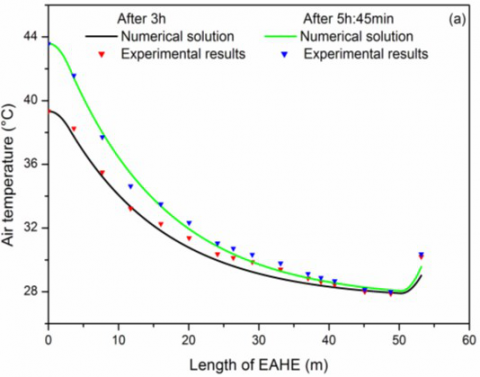## 6.2.png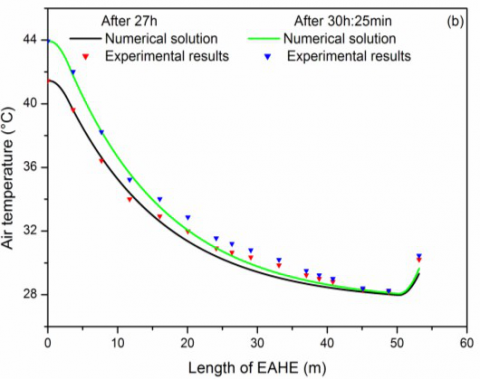## 6.3.png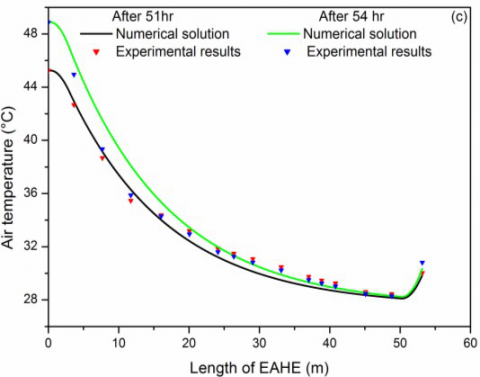## 6.4.png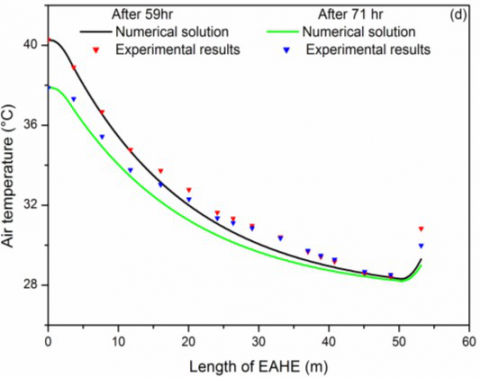Figure 6. Air temperatures comparison between numerical solutions and experimental results: figs (a), (b), (c) and (d).

## 7.png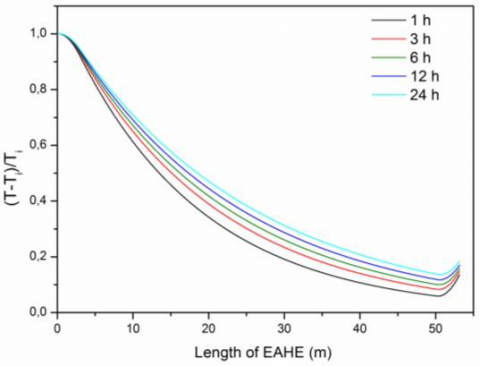Figure 7. Graph of the present numerical solutions.

## 8.pngFigure 8. Values of air temperatures in different sections of the EAHE over time.

## 9.1.png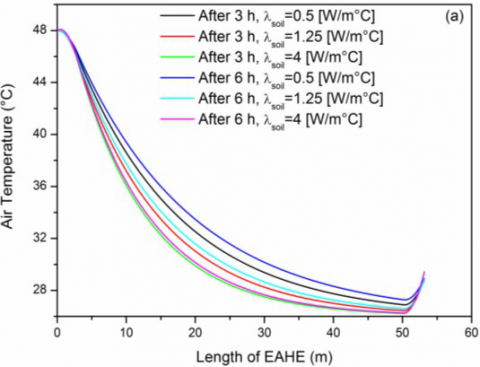## 9.2.png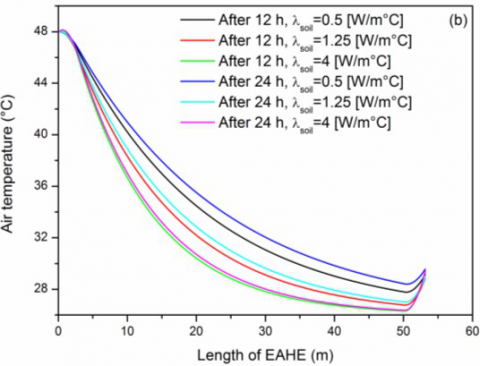Figure 9. Hourly variation of air temperature along the EAHE for different soil thermal conductivities.

## 10.1.png## 10.2.png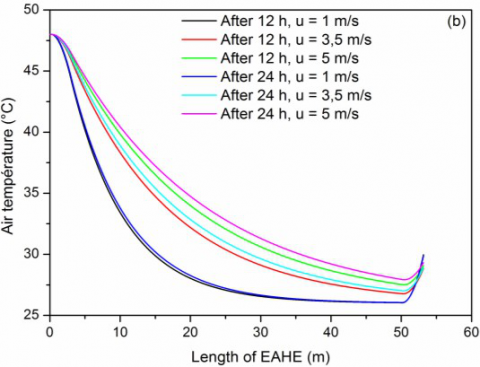Figure 10. Hourly variation of air temperature along the EAHE for different air flow velocities.

## 11.png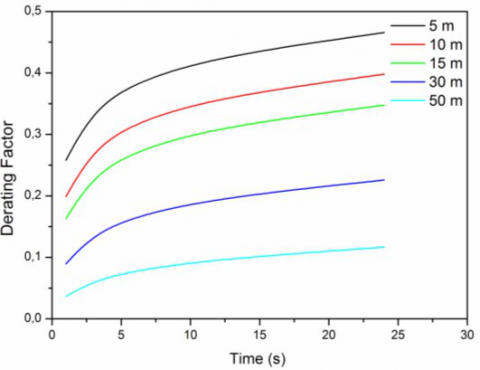Figure 11. Variation in derating factor in different sections of the EAHE over time.

## 12.pngFigure 12. Variation of efficiency over time.

5. Conclusion

In this work, we presented in a detailed manner an experimental and numerical study of the air cooling using an earth air heat exchanger (EAHE). A transient one- dimensional numerical model using finite differences method is proposed to determine the temperature distribution along the pipe. The model was compared with experimental measurements performed in the University of Biskra for three days in continuous operation mode. Maximum air temperature drop and maximum thermal efficiency of 18.06 °C and 78.96 % respectively were achieved for high inlet air temperature (48.87°C). The validation between numerical and experimental results shows a fair agreement with a maximum relative error of 7.46%.

From this study the main concluding remarks are as follows: i) the Exploitation of the EAHE alone cannot give the thermal comfort of cooling. Consequently, it can reduce the electric power consumption like using the means of air conditioning. ii) in order that, the flowing air preserves its cool most possible in the vertical portion III, it must be ensure sufficient conditions of thermal insulation in this portion, iii) the continuous operation mode does not affect the thermal performances and outlet air temperature of the EAHE during all 71 h of operating for high soil thermal conductivities and low air flow velocities and iv) the initial 30 m of the pipe provides almost 78% of the total air temperature drop.

Acknowledgments

The authors thank the University of Biskra for supporting the experimental research and the Laboratory of Mechanical Engineering LGM for financial support. We also thank Mebarek BAHRI for his contribution in writing this paper.

Nomenclature
 Tsoil soil temperature, °C Ta air temperature inside the EAHE, °C Tai inlet air temperature, °C Ta1 outlet air temperature at the vertical portion I, °C Ta2 outlet air temperature at the horizontal portion II, °C Ti soil temperature at depth δ, °C u air velocity inside the pipe, m.s-1 h convective heat transfer coefficient h, w.m-2.°C -1 x Longitudinal coordinate, m z vertical coordinate, m r1 inner radius of the pipe, m r2 thickness of the pipe, m r3 adiabatic layer radius of the soil, m l length, m s exchange surface, m2 R thermal resistance, m.k.w-1 m air mass, kg cpair specific heat, j.kg-1.°C -1 t Time, s Greek symbols λsoil soil thermal conductivity, w.m-1.°C -1 α soil thermal diffusivity, m2.s-1 δ penetration depth, m ρ air density, kg.m-3 φ heat flow, watt Abbreviations EAHE Earth air heat exchanger
References

 Scott N., Parsons R., Kochler T. (1965). Analysis and performance of an earth-air heat exchanger.

 Goswami D.Y., Ileslamlou S. (1990). Performance analysis of a closed-loop climate control system using underground air tunnel, Journal of Solar Energy Engineering, Vol. 112, No. 2, pp. 76-81. DOI:10.1115/1.2929650

 Bahadori M.N. (1978). Passive cooling systems in Iranian architecture, Scientific American, Inc., Vol.238, No. 2, pp. 144-150,152,154. DOI:10.1038/scientificamerican0278-144

 Bisoniya T.S., Kumar A., Baredar P. (2013).Experimental and analytical studies of earth–air heat exchanger (EAHE) systems in India: a review,Renewable and Sustainable Energy Reviews, Vol. 19 pp. 238-246. DOI: 10.1016/j.rser.2012.11.023

 Pfafferott J. (2003). Evaluation of earth-to-air heat exchangers with a standardised method to calculate energy efficiency, Energy and Buildings, Vol. 35 10,pp. 971-983. DOI: 10.1016/S0378-7788(03)00055-0

 Trombe A., Pettit M., Bourret B. (1991). Air cooling by earth tube heat exchanger: Experimental approach,Renewable Energy, Vol. 1, No. 5, pp. 699-707,. DOI:10.1016/0960-1481(91)90016-I

 Thanu N.M., Sawhney R.L., Khare R.N., Buddhi D.(2001). An experimental study of the thermal performance of an earth-air-pipe system in single pass mode, Solar Energy, Vol. 71, No. 6, pp. 353-364.DOI: 10.1016/S0038-092X(01)00072-X

 Ghosal M.K., Tiwari G.N. (2006). Modeling and parametric studies for thermal performance of an earth to air heat exchanger integrated with a greenhouse, Energy Conversion and Management, Vol. 47, No. 13–14, pp. 1779-1798. DOI:10.1016/j.enconman.2005.10.001

 Ghosal M.K., Tiwari G.N., Srivastava N.S.L. (2004).Thermal modeling of a greenhouse with an integrated earth to air heat exchanger: an experimental validation,Energy and Buildings, Vol. 36, No. 3, pp. 219-227,2004. DOI: 10.1016/j.enbuild.2003.10.006.

 Shukla A., Tiwari G.N., Sodha M.S. (2006). Thermal modeling for greenhouse heating by using thermal curtain and an earth–air heat exchanger, Building and  Environment, Vol. 41, No. 7, pp. 843-850. DOI:10.1016/j.buildenv.2005.04.014

 Sodha M.S., Sharma A.K., Singh S.P., Bansal N.K.,Kumar A. (1985). Evaluation of an earth—air tunnel system for cooling/heating of a hospital complex,Building and Environment, Vol. 20, No. 2, pp. 115-122. DOI: 10.1016/0360-1323(85)90005-8.

 Moummi N., Benfatah H., Hatraf N., Moummi A.,Youcef-Ali S. (2010). Le rafraîchissement par lagéothermie: étude théorique et expérimentale dans lesite de Biskra, Revue des Energies Renouvelables, Vol. 13, No. 3, pp. 399-406.

 Trombe A., Serres L. (1994). Air-earth exchanger study in real site experimentation and simulation,Energy and Buildings, Vol. 21 2, pp. 155-162. DOI:10.1016/0378-7788(94)90008-6.

 Krarti M., Kreider J.F. (1996). Analytical model for heat transfer in an underground air tunnel, Energy Conversion and Management, Vol. 37, No. 10, pp.1561-1574. DOI: 10.1016/0196-8904(95)00208-1

 Hollmuller P. (2003). Analytical characterisation of amplitude-dampening and phase-shifting in air/soil heat-exchangers, International Journal of Heat and Mass Transfer, Vol. 46, No. 22, pp. 4303-4317. DOI:10.1016/S0017-9310(03)00199-6

 Rouag A., Benchabane A., Labed A., Belhadj K.,Boultif N. (2016). Applicability of a solar adsorption cooling machine in semiarid regions: proposal of supplementary cooler using earth-water heat exchanger, International Journal of Heat and Technology, Vol. 34, No. 2, pp. 281-286. DOI:10.18280/ijht.340218

 Mihalakakou G., Santamouris M., Asimakopoulos D.,I. Tselepidaki (1995). Parametric prediction of the buried pipes cooling potential for passive cooling applications, Solar Energy, Vol. 55, No. 3, pp. 163-173. DOI: 10.1016/0038-092X(95)00045-S

 Lee K.H., Strand R.K. (2008). The cooling and heating potential of an earth tube system in buildings,Energy and Buildings, Vol. 40, No. 4, pp. 486-494,2008. DOI: 10.1016/j.enbuild.2007.04.003

 Brum R.S., Vaz J., Rocha L.A.O., dos Santos E.D.,Isoldi L.A. (2013). A new computational modeling to predict the behavior of Earth-air heat exchangers,Energy and Buildings, Vol. 64, pp. 395-402. DOI:10.1016/j.enbuild.2013.05.032

 Vaz J., Sattler M.A., dos Santos E.D., Isoldi L.A.(2011). Experimental and numerical analysis of an earth–air heat exchanger, Energy and Buildings, Vol.43, No. 9, pp. 2476-2482. DOI:10.1016/j.enbuild.2011.06.003

 Thiers S., Peuportier B. (2008). Thermal and environmental assessment of a passive building equipped with an earth-to-air heat exchanger in France, Solar Energy, Vol. 82, No. 9, pp. 820-831.DOI: 10.1016/j.solener.2008.02.014

 Barakat S., Ramzy A., Hamed A.M., El Emam S.H.(2016). Enhancement of gas turbine power output using earth to air heat exchanger (EAHE) cooling system, Energy Conversion and Management, Vol.111, pp. 137-146. DOI:10.1016/j.enconman.2015.12.060

 Ozgener O., Ozgener L., Goswami D.Y. (2011).Experimental prediction of total thermal resistance of a closed loop EAHE for greenhouse cooling system,International Communications in Heat and Mass Transfer, Vol. 38, No. 6, pp. 711-716. DOI:10.1016/j.icheatmasstransfer.2011.03.009

 Ozgener L. (2011). A review on the experimental and analytical analysis of earth to air heat exchanger(EAHE) systems in Turkey, Renewable and Sustainable Energy Reviews, Vol. 15, No. 9, pp.4483-4490. DOI: 10.1016/j.rser.2011.07.103

 Claesson J., Dunand A. (1983). Heat extraction from the ground by horizontal pipes: a mathematical analysis, BFR Swedish Council for Building Research.

 Bansal V., Misra R., Agarwal G.D., Mathur J. (2013).Transient effect of soil thermal conductivity and duration of operation on performance of Earth Air Tunnel Heat Exchanger, Applied Energy, Vol. 103, pp.1-11. DOI: 10.1016/j.apenergy.2012.10.014

 Nilsson C.E. (1991). Preheating of ambient air by a system of earth tubes as a heat source for buildings,Chalmers University of Technology.

 Mihalakakou G., Lewis J.O., Santamouris M., On the heating potential of buried pipes techniques —application in Ireland, Energy and Buildings, Vol. 24,No. 1, pp. 19-25, 1996. DOI: 10.1016/0378-7788(95)00957-4

 Bojic M., Trifunovic N., Papadakis G., Kyritsis S.(1997). Numerical simulation, technical and economic evaluation of air-to-earth heat exchanger coupled to a building, Energy, Vol. 22, No. 12, pp.1151-1158. DOI: 10.1016/S0360-5442(97)00055-8

 Chel A., Janssens A., de Paepe M. (2015). Thermal performance of a nearly zero energy passive house integrated with the air–air heat exchanger and the earth–water heat exchanger, Energy and Buildings, Vol. 96, pp. 53-63. DOI:10.1016/j.enbuild.2015.02.058

 Soni S.K., Pandey M., Bartaria V.N. (2015). Ground coupled heat exchangers: A review and applications,Renewable and Sustainable Energy Reviews, Vol. 47pp. 83-92. DOI: 10.1016/j.rser.2015.03.014

 De Jesus Freire A., Alexandre J.L.C., Silva V.B.,Couto N.D., Rouboa A. (2013). Compact buried pipes system analysis for indoor air conditioning, Applied Thermal Engineering, Vol. 51, No. 1-2, pp. 1124-1134. DOI: 10.1016/j.applthermaleng.2012.09.045

 Ozisik M.N. (1993). Heat Conduction, 2nd Edition,John Wiley & Sons.

 Al-Khoury R. (2011). Computational Modeling of Shallow Geothermal Systems, CRC Press.

 De Jesus Freire A., Alexandre J.L.C., Silva V.B.,Couto N.D., A. Rouboa (2013). Compact buried pipes system analysis for indoor air conditioning, Applied Thermal Engineering, Vol. 51, No. 1, pp. 1124-1134.DOI: 10.1016/j.applthermaleng.2012.09.045

 Diersch H.J.G., Bauer D., Heidemann W., Rühaak W.,Schätzl P. (2011). Finite element modeling of borehole heat exchanger systems: Part 2. Numerical simulation, Computers & Geosciences, Vol. 37, No. 8,pp. 1136-1147. DOI: 10.1016/j.cageo.2010.08.002

 Mnasri T., Younès R.B. (2010). Modeling of unsteady turbulent flow in a buried co-axial exchanger—the use of Green's functions theory, Numerical Heat Transfer, Part A: Applications, Vol. 58, No. 12, pp.963-983. DOI: 10.1080/10407782.2010.529031

 Philippe M., Bernier M., Marchio D. (2009). Validity ranges of three analytical solutions to heat transfer in the vicinity of single boreholes, Geothermics, Vol. 38,No. 4, pp. 407-413. DOI:10.1016/j.geothermics.2009.07.002

 Belloufi Y., Brima A., Atmani R., Moummi N.,Aissaoui F. (2016). Etude theorique et experimentaledu rafraichissement de l’air par un echangeur geothermique AIR/SOL, LARHYSS Journal, Vol. 25,pp. 121-137.

 Aissaoui F., Benmachiche A.H., Brima A., Bahloul D., Belloufi Y. (2016). Experimental and Theoretical Analysis on Thermal Performance of the flat plate solar air collector, International Journal of Heat and Technology, Vol. 34, No. 2, pp. 213-220. DOI:10.18280/ijht.340209

 V. Bansal, R. Misra, G. D. Agarwal and J. Mathur,‘Derating Factor’ new concept for evaluating thermal performance of earth air tunnel heat exchanger: Atransient CFD analysis, Applied Energy, vol. 102 pp.418-426, 2013. DOI: 10.1016/j.apenergy.2012.07.027.

 Belloufi Y., Brima A., Atmani R., Moummi N.,Aissaoui F. (2016). Etude theorique et experimentaledu rafraichissement de l’air par un echangeur geothermique AIR/SOL, LARHYSS Journal, Vol. 25,pp. 121-137.

 Aissaoui F., Benmachiche A.H., Brima A., Bahloul D., Belloufi Y. (2016). Experimental and Theoretical Analysis on Thermal Performance of the flat plate solar air collector, International Journal of Heat and Technology, Vol. 34, No. 2, pp. 213-220. DOI:10.18280/ijht.340209